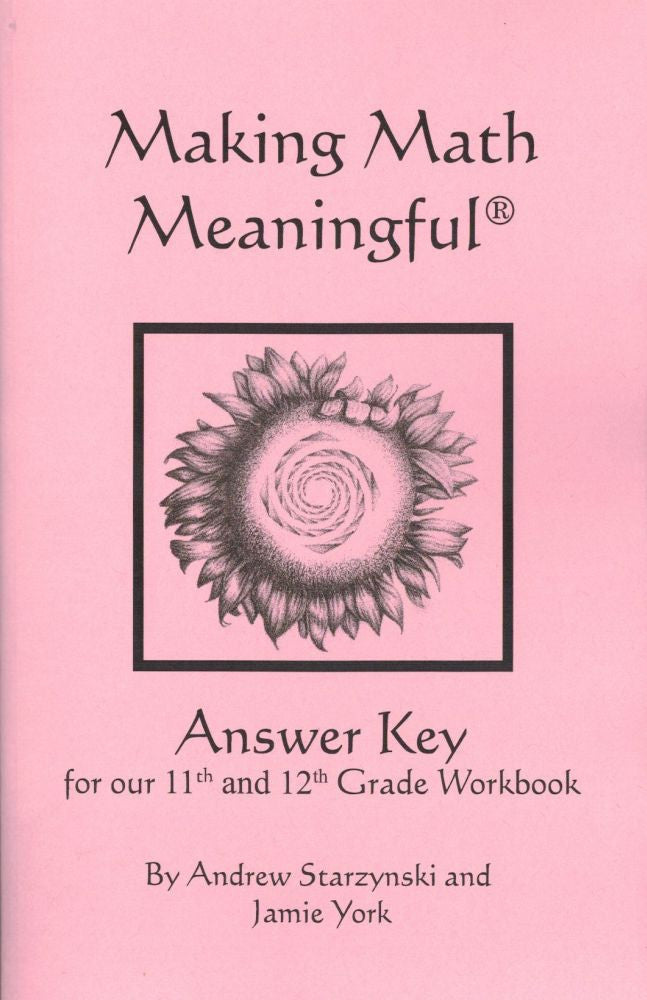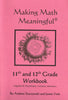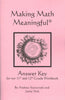\$ 6.00

Making Math Meaningful: An 11th and 12th Grade Workbook (Algebra II, Precalculus and some Calculus) by Andrew Starzynski and Jamie York.

Through a year and a half of study, this workbook weaves together Cartesian geometry, trigonometry, complex numbers, and other key topics found in traditional algebra II and precalculus courses, and ends with two great units of calculus. Any student completing this book is poised to study anything in college!

The units covered in this workbook are:

• Cartesian Geometry, Part I
• Cartesian Geometry, Part II
• Cartesian Geometry, Part III
• Cartesian Geometry, Part IV
• Logarithms, Part III
• Trigonometry, Part II
• Trigonometry, Part IV
• Complex Numbers, Part I
• Complex Numbers, Part II
• Possibility & Probability, Part II
• Calculus, Part II
• Problem Solving Exercises

Workbook: 5.5" x 8.5", 134 pages, illustrated, lay-flat, saddle-stitched, soft-cover

Workbook and Answer Key sold separately.